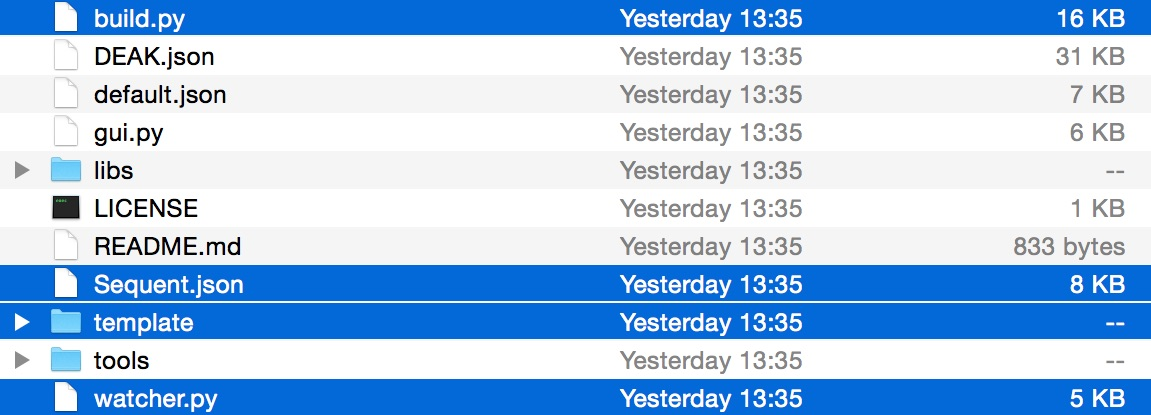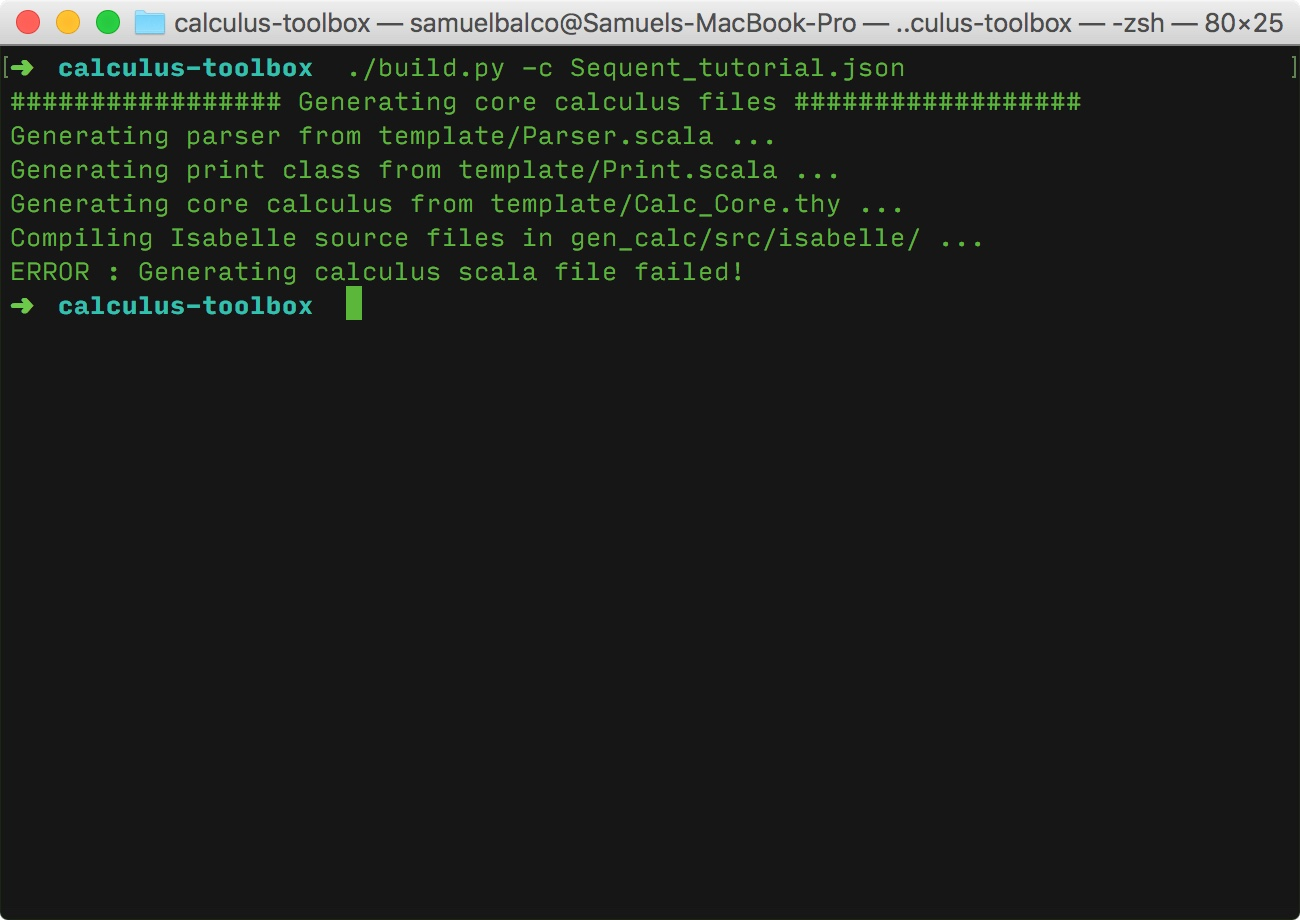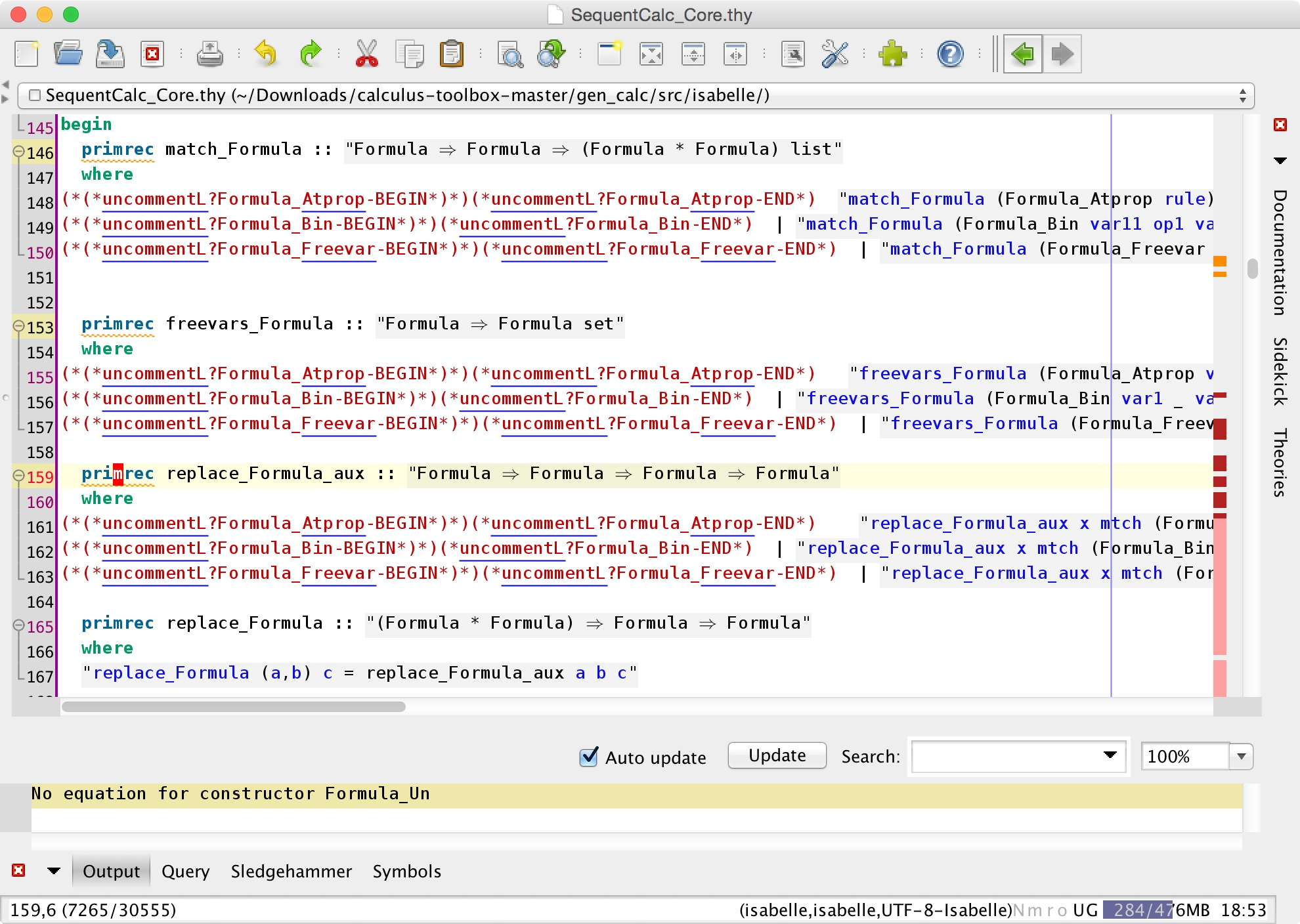# Building a Sequent Calculus Toolbox - Part 2

This article is the second part of a tutorial on building a sequent calculus toolbox in Isabelle and Scala. If you have not yet read part one, you can find it here. Part three is also now available.

This part of the tutorial will focus on the process of compiling the Seqeunt_tutorial.json file we created previously. I will demonstrate the build script and supporting tools, which allow for an easy extension and modification of an existing calculus into a new one. To see the final result, i.e. the compiled version of the Sequent calculus toolbox, download or clone the Sequent-calculus-tool repository.

In order to get started, we need to clone or download a copy of the calculus-toolbox, which contains all the necessary tools to build our Sequent calculus toolbox. (Note that the changes I will be making to the files in this section have already been applied to the templates in the calculus-toolbox repository.)

To avoid unnecessary confusion, I will refer to the toolbox used to generate the Sequent calculus Isabelle and Scala files, as the calculus-toolbox (I am going to try to use the italics consistently). This is sort of a meta-toolbox, used for generating other calculus toolboxes. When I talk about a calculus toolbox, I will usually refer to our Sequent calculus toolbox we will be generating in this section, using the meta-toolbox.

Before we start building the Sequent calculus toolbox, here is a brief overview of the essential files and folders in the calculus-toolbox (the meta tool, remember), which are highlighted below.#### build.py

This is the main build script used to generate the Sequent calculus toolbox. To get a better idea of what the build script does, head over to the docs on code generation.

#### Sequent.json

Sequent.json is the calculus description file we created in the previous part of this tutorial.

#### template/

The calculus-toolbox, namely the build script, can be thought of as a macro expander. Broadly, the build script takes in a JSON file and a set of templates and replaces the information in the file into the templates, thus generating the custom calculus toolbox. This section will detail some of the modifications of the template files that will be needed for the sequent calculus.

#### watcher.py

This is a small utility which makes it easier to modify the template files. More information on its use can be found in the docs.

## Compiling the calculus - first attempt

To demonstrate the work-flow of modifying an existing calculus toolbox (referring not to the calculus-toolbox meta-toolbox, rather to the files the calculus-toolbox produces) to build a new one, we will start with a template folder containing the files necessary to build DCPL (display classical propositional logic), formalized in default.json. To follow this tutorial, replace the default /template folder in the calculus-toolbox repository you cloned/downloaded, with the version above. If you have been following the tutorial and created your own version of the JSON calculus description file for our Sequent calculus, copy this JSON file to the calculus-toolbox repository. Alternatively, you can download this file here.

As a naive first attempt, we can try compiling our Sequent calculus by using the template files of the DCPL. To do this, run the following command in terminal (inside the calculus-toolbox folder):

./build.py -c Sequent_tutorial.json

You should be quickly greeted with the following error message:When we look back into the calculus-toolbox folder, there is a new folder called gen_calc. From the script error message, we know that the build failed at compiling files in gen_calc/src/isabelle/

The folder should contain two files: ROOT and SequentCalc_Core.thy. It is the second one we will have to modify to work with our Sequent calculus.

## Troubleshooting in the Isabelle IDE

At this point, we will have to open the SequentCalc_Core.thy file in Isabelle to find out what kind of an error caused the script to fail (and fix it). If you have not installed Isabelle yet, head over to the Isabelle homepage, download and install the latest version (this tutorial uses the 2015 version).

Before we start editing the SequentCalc_Core.thy, it is a good time to introduce the watcher.py script and explain its purpose.

To do that, I need to explain the motivation behind the calculus-toolbox as such. The main component of the calculus-toolbox is the build script, which takes in our JSON description file, containing the terms and the rules of a calculus, and expands this concise definition into multiple Isabelle theories and Scala files. These files are stored in the templates folder and contain "holes", which are filled with the information in the JSON file.

Due to this centralized definition of the calculus, adding rules or logical connectives becomes much easier, as such modifications to the compiled calculus would mean making tedious and error-prone changes to multiple Isabelle and Scala files.

However, not all definitions are automatically generated by the build script, and, as is the case in this tutorial, we might want to step in and manually modify the calculus. We therefore want any manual changes made to the calculus to propagate back into the templates so that modifying the calculus on a high level, such as adding or removing rules, remains centralized (i.e. is done using the build script and the JSON description file).

This propagation of changes back to the templates is precisely what the watcher utility does. When running in the background, it monitors the source folder of the compiled calculus and propagates any changes made to its files back to the corresponding templates. It does this by decompiling the source files back into template files each time these are modified.

To run the watcher script, open a new terminal window inside calculus-toolbox and run the following command (the flags used in the command are explained here):

./watcher.py -s gen_calc/src -d template -i "scala/SequentCalc.scala, .thy#, .thy~" -r "scala/ > , isabelle/ > , SequentCalc_Core.thy > Calc_Core.thy, SequentCalc.thy > Calc_Rules.thy"


Opening the SequentCalc_Core.thy file and letting the continuous checker process the file, we run into an error in the lemma freevars_replace_Formula_simp on line 283:

Failed to finish proof:
goal (1 subgoal):
1. ⋀x1a a.
(free ∈ freevars a ⟹ replace (free, free) a = a) ⟹
free ∈ freevars (U⇩F x1a a) ⟹ replace (free, free) (U⇩F x1a a) = U⇩F x1a a


To some, this error might be readable, to others it might be utter garbage. I will therefore try to explain why it arises and how to fix it.

The lemma tries to prove the following statement:

free ∉ freevars (a::Atprop) ⟶ replace (free,free) a = a


Loosely translated to English, this says:

For any free variable not found in the formula 'a', replacing the free variable with itself in 'a' yields 'a'.

Since this lemma tries to prove a property of replace, it is logical to first look at its definition in the file.As one might begin to suspect from the warning shown above, the root of this error arises from one of the points of difference between the Sequent calculus and DCPL (since we are using the template files of DCPL), mentioned in the first part of this tutorial:

• The formulae of the template calculus don't include the unary $$\lnot$$ operator

The definition of Formula in SequentCalc_Core.thy includes a Formula_Un constructor as we have defined it in the JSON file, whereas DCPL does not contain any unary connectives in its formulas.

As the warning states, the functions match_Formula, freevars_Formula and replace_Formula_aux all lack a constructor for Formula_Un. In the next section, I briefly outline the purpose of each of these functions and show the appropriate code that needs to be added to each function.

### Fixing the match, replace and freevars functions

#### match_Formula

As the signature of the match function suggests, this function takes in two formulas and creates a list of pairs of matched substructures.

The first argument of match_Formula is a formula template, which is a formula that may contain free variables at its leaves.
The second argument is a formula that does not contain any free variables. This formula is matched against the template and decomposed into pairs of free variables of the template formula and the matched substructures of the second formula.
(Note that these conditions are not actually enforced in this function, rather, they must be enforced by any function that uses match_Formula, in order to assure the correct behavior of match_Formula)

To add a constructor case for Formula_Un we first take a look at the Formula_Bin constructor:

(*(*uncommentL?Formula_Bin-BEGIN*)*)(*uncommentL?Formula_Bin-END*)
| "match_Formula (Formula_Bin var11 op1 var12) x =
(case x of
(Formula_Bin var21 op2 var22) ⇒
(if op1 = op2 then
(match var11 var21) @m (match var12 var22)
else [])
| _ ⇒ [])"
(*uncommentR?Formula_Bin-BEGIN*)(*(*uncommentR?Formula_Bin-END*)*)


Since Formula_Un is made up of a unary connective and another forumula, the match has to simply check if the unary connectives of the two formulas match and if they do, recursively call match on the formulas that they contain.

(*(*uncommentL?Formula_Un-BEGIN*)*)(*uncommentL?Formula_Un-END*)
| "match_Formula (Formula_Un op1 var1) x =
(case x of
(Formula_Un op2 var2) ⇒
(if op1 = op2 then
(match var1 var2)
else [])
| _ ⇒ [])"
(*uncommentR?Formula_Un-BEGIN*)(*(*uncommentR?Formula_Un-END*)*)


#### freevars_Formula

This function simply returns all the free variables the given formula contains. The code for the Formula_Un constructor is fairly straight forward:

(*(*uncommentL?Formula_Un-BEGIN*)*)(*uncommentL?Formula_Un-END*)
| "freevars_Formula (Formula_Un _ var) = (freevars var)"
(*uncommentR?Formula_Un-BEGIN*)(*(*uncommentR?Formula_Un-END*)*)


#### replace_Formula

This function can be thought of as the reverse of the match function. As its type signature shows, replace_Formula takes in a pair of formulas and a template formula (as the second argument) and returns another formula. What the function does is substitute the first formula of the pair by the second formula of the pair in the template formula, if the first formula of the pair occurs anywhere within the template formula. If this sounds confusing, here is an example:

If we have a pair $$(?X, A \vee B)$$, where $$?X$$ is a free variable, and a template formula $$?X \land (?Y \land ?X)$$, passing these two arguments to the replace function will yield the formula: $$(A \vee B) \land (?Y \land (A \vee B))$$

However, when we take a closer look at replace_Formula, we can see that it is only a wrapper function for an auxiliary function replace_Formula_aux. (The reason for having this auxiliary function is not at all obvious and won't be discussed in this tutorial.)

Without further ado, here is the missing code for Formula_Un:

(*(*uncommentL?Formula_Un-BEGIN*)*)(*uncommentL?Formula_Un-END*)
| "replace_Formula_aux x mtch (Formula_Un op1 var) =
Formula_Un op1 (replace_Formula_aux x mtch var)"
(*uncommentR?Formula_Un-BEGIN*)(*(*uncommentR?Formula_Un-END*)*)


In all the above functions, the snippets included a rather ugly set of comments surrounding the code:

(*(*uncommentL?Formula_Un-BEGIN*)*)(*uncommentL?Formula_Un-END*)
...
(*uncommentR?Formula_Un-BEGIN*)(*(*uncommentR?Formula_Un-END*)*)


The reason for keeping these comments is to do with the way the templates work. By surrounding each block of code corresponding to a certain constructor with these special matching comment blocks, we can easily remove the Formula_Un constructor in the future and not have to come back to this file to delete the code we have just added, because it will stay commented out in the absence of a Formula_Un constructor in the JSON description file.

### Fixing the lemmas

Now that we have amended the definitions of match, replace and freevars, we need to go back to the initial error in the freevars_replace_Formula_simp lemma and add the missing proof for Formula_Un.

As this proof is trivial, we simply copy the proof block for Formula_Bin and modify it accordingly:

(*(*uncommentL?Formula_Un-BEGIN*)*)(*uncommentL?Formula_Un-END*)
case (Formula_Un c x) thus ?case by simp
next
(*uncommentR?Formula_Un-BEGIN*)(*(*uncommentR?Formula_Un-END*)*)


Next error is in the lemma freevars_replace_Formula_simp2. Copying and modifying the proof for Formula_Bin, we obtain:

(*(*uncommentL?Formula_Un-BEGIN*)*)(*uncommentL?Formula_Un-END*)
case (Formula_Un c x)
have 1: "free ∈ freevars (Formula_Un c x) ⟶ replace (free, free) x = x" using Formula_Un.hyps by auto
have "free ∈ freevars (Formula_Un c x) ⟶ replace (free, free) (Formula_Un c x) = Formula_Un c (replace (free, free) x)" by auto
with 1 show ?case by (metis Formula_Un.prems)
next
(*uncommentR?Formula_Un-BEGIN*)(*(*uncommentR?Formula_Un-END*)*)


In the next lemma, match_Formula_simp, the proof for Formula_Un is trivial again:

(*(*uncommentL?Formula_Un-BEGIN*)*)(*uncommentL?Formula_Un-END*)
case (Formula_Un c x) thus ?case by auto
next
(*uncommentR?Formula_Un-BEGIN*)(*(*uncommentR?Formula_Un-END*)*)


Finally, we add the proof below to the lemma inv_Formula:

(*(*uncommentL?Formula_Un-BEGIN*)*)(*uncommentL?Formula_Un-END*)
case (Formula_Un c x)
obtain z where 0: "z = (Formula_Un c x)" by simp
then have 1: "∀free ∈ set (match z z). replace free z = Formula_Un c (replace free x)"
by auto
have "∀free ∈ set (match z z). replace free x = x" using "0" Formula_Un.hyps by auto
thus ?case by (metis "0" "1")
next
(*uncommentR?Formula_Un-BEGIN*)(*(*uncommentR?Formula_Un-END*)*)


Once all of these proofs are fixed, there should be no more error messages. If this is the case, we can save SequentCalc_Core.thy and try running the build script again.

## Compiling the calculus - second attempt

If everything has been done correctly, after running the build script again, we should be greeted with the message:

SequentCalc has been successfully built...

If this is the case, navigate to the gen_calc folder and run:

./build.py

Rather confusingly, this build.py is a different build script to the calculus-toolbox one! This build script compiles the UI of our Sequent calculus toolbox. If this build script finishes without any error messages, we can launch the UI by running:

make gui

## One more thing

Before I conclude part two of this tutorial, there is one more modification that we need to do to the template files. Even though the calculus was generated and compiled successfully, there is one more function definition in the template files that needs to be amended due to the introduction of Formula_Un in our calculus. More specifically, we need to add a constructor for Formula_Un to the function rulifyFormula in the file SequentCalc.thy, found in the /src/isabelle/ folder of our compiled calculus (for reference, here is the fully compiled version from the Sequent-calculus-tool repository).

Add the following definition, making sure the watcher utility is running, so that this change is carried back to the template files:

(*(*uncommentL?Formula_Un-BEGIN*)*)(*uncommentL?Formula_Un-END*)
"rulifyFormula (Formula_Un c y) = (Formula_Un c (rulifyFormula y))" |
(*uncommentR?Formula_Un-BEGIN*)(*(*uncommentR?Formula_Un-END*)*)


We can now simply run the build script inside our generated calculus (gen_calc) folder (not the main build script for generating the calculus...confusing I know), which will recompile the Scala classes of the Sequent calculus toolbox UI.

Part three of this tutorial is now available.